# planning algorithms chapter 2 :Discrete Planning

## 离散可行规划导论

### 问题定义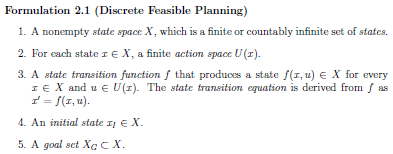1. 非空状态空间 X
2. 对于每个状态 x，存在一个有限的动作空间 U(x)
3. 对于每个状态和动作空间，存在状态转移方程，产生一个新的状态
4. 一个初始状态 xi
5. 一个目标集 Xg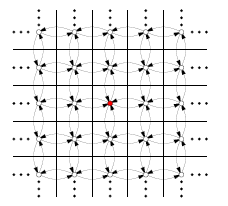### 离散规划的例子

• 2D 网格上移动（“迷宫”）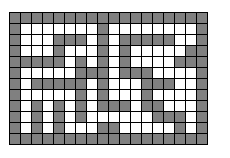• 魔方拼图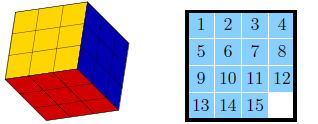## 图搜索算法

### 前向搜索算法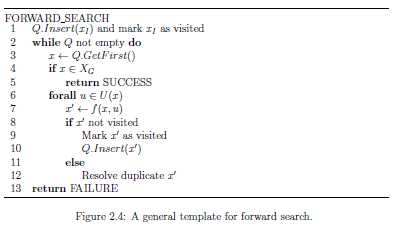• 广度优先：FIFO
• 深度优先：LIFO
• Dijkstra ：一种图单源最短路径搜索算法，一种特殊的动态规划形式
在Dijkstra中，图上每条边附带一个代价（l(x, u) >= 0），Q 内部是按照从初始状态到达该状态的累计代价（C(x),cost-to-come）排序。cost-to-come 在搜索过程中通过DP方式来增量计算（C(x‘) = C*(x) + l(x, u), * 代表最优)。
Dijkstra 可以保证一旦某个状态被访问，则该状态的 cost-to-come一定是最优的。Dijkstra 内部 Q 实现采用的 Fibonacci heap 这种数据结构，可以实现在常数时间内判断某个状态是否被访问过。
• A-star ：基于Dijkstra进行扩展，引入启发项值（G(x)，cost-to-go)，当G(x) = 0 时， A-star 退化成Dijkstra，Q 内部是按照从初始状态到达目标状态的预估最优代价( C(x’) + G(x‘)) 进行排序。
• 最佳优先搜索：Q 内部是按照 cost-to-go 排序，一种贪心搜索，不保证最优，但搜索速度快。
• 迭代加深搜索：通过不断增加深度优先搜索深度的一种搜索，将深度优先搜索转换为一种系统性搜索方式（能够访问可到达的所有状态)。
迭代加深搜索相比 BFS 使用更少的内存，迭代加深搜索结合 A-star 的思想，形成了 IDA* 算法，在每次迭代过程中，最大深度步长为C(x’) + G(x‘)。

### 其他搜索算法

• 反向搜索算法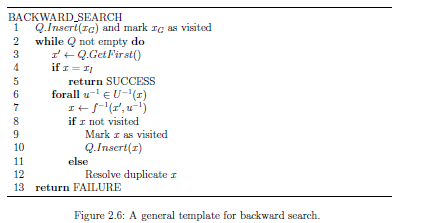• 双向搜索算法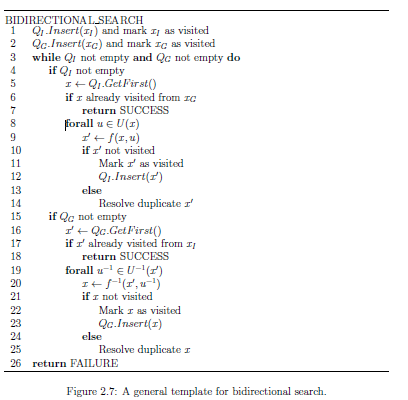### 搜索算法的统一视角

1. 初始
搜索开始时，搜索图 G(V,E)中 E为空集，V只包含初始状态
2. 选择顶点
从V中选择一个顶点，这通常是通过维护一个优先级队列实现
3. 应用动作
基于V中选择的某个顶点，应用动作后，生成一个新的状态 x = f(x0, u)
4. 向搜索图中插入有向边
新状态 x 如果不在 V 中，则将 x 插入到 V 中
5. 检查解决方案
如果只有一颗搜索树，根据搜索图 G 得到从初始状态到目标状态的路径会比较简单。如果搜索树数量大于 1 颗，复杂度会增加。
6. 返回到步骤 2
迭代直到找到一个解决方案。

## 离散最优规划

### 最优定长规划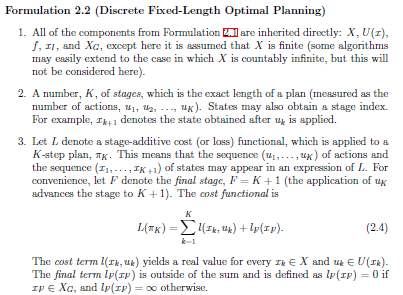$L\left ( \pi _{K} \right ) = \sum_{k=1}^{K}l(x_{k},u_{k}) + l_{F}(x_{F})$

#### 反向值迭代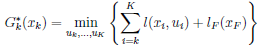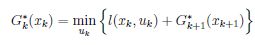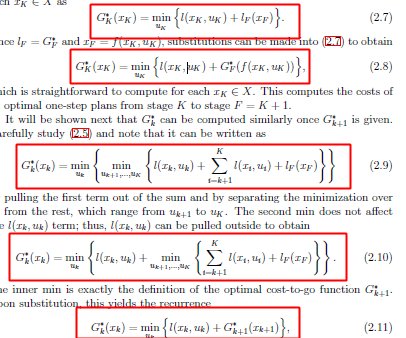$G_{F}^{ \ast}\rightarrow G_{K}^{ \ast}\rightarrow G_{K-1}^{ \ast}\cdots G_{k}^{ \ast}\rightarrow G_{k-1}^{ \ast}\rightarrow\cdots G_{2}^{ \ast}\rightarrow G_{1}^{ \ast}$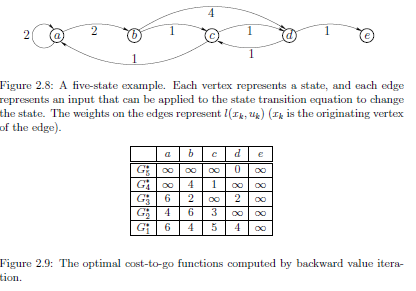#### 正向值迭代

• 为什么需要正向值迭代？正向值和反向值迭代的区别是什么？
反向：
反向值迭代可以同时找到各顶点到目标顶点的最优计划；
反向值迭代需要目标顶点是确定不变的；
正向：
正向值迭代可以用来找到从初始顶点出发到其他各顶点的最优计划；
正向值迭代需要初始顶点是确定不变的；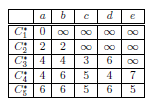### 最优不定步长规划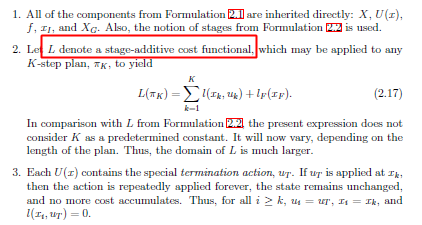$L\left ( \pi _{K} \right ) = \sum_{k=1}^{K}l(x_{k},u_{k}) + l_{F}(x_{F})$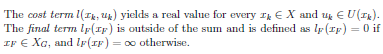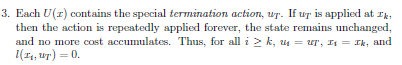## 使用逻辑定义离散规划

### STRIPS-Like 表示法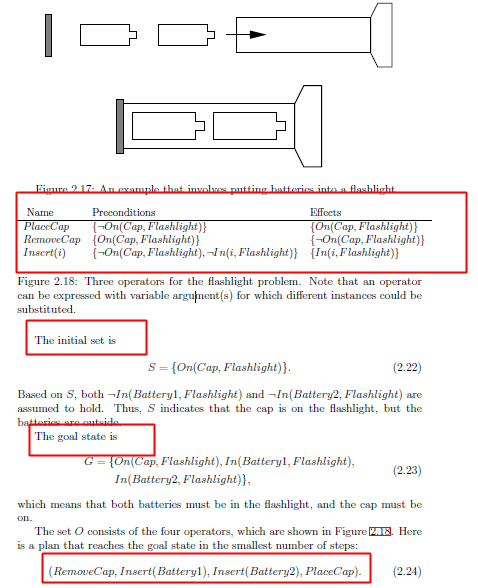posted @ 2019-07-03 16:23  不燥不怕  阅读(...)  评论(...编辑  收藏